Nov 2015

I will post seven new problems every weekend, one for each day of the week. The idea is that you work on your own during the week and I will post answers on the morning of the following Saturday.

For instructions on submitting weekly answers, click on the “Logistics” tab.
For previously posted problems, click on the “Archive” tab.
For previous prizes, click on the “News” tab.

Prizes:  As encouragement, JerseySTEM is offering prizes to recognize those who have consistently answered questions correctly. The next round of prizes will be given out Friday Nov 20.  The prizes cover the 5 weeks of Oct 17 – Nov 20, and I will pick the best 4 out of the 5 week for each of you.

Week of Nov 14 – Nov 20: This week’s problems are calculation puzzles. Click GoogleForm to submit answers.

Answers: A, A, D, D, E, B, E

Nov 14: The operation$\otimes$ is defined for all nonzero numbers by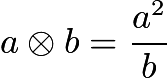$a\otimes b = \dfrac{a^2}{b}$. Determine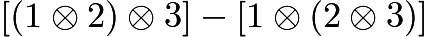$[(1\otimes 2)\otimes 3] - [1\otimes (2\otimes 3)]$.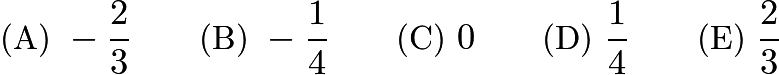$\text{(A)}\ -\dfrac{2}{3} \qquad \text{(B)}\ -\dfrac{1}{4} \qquad \text{(C)}\ 0 \qquad \text{(D)}\ \dfrac{1}{4} \qquad \text{(E)}\ \dfrac{2}{3}$

Source: AMC8 2000

Nov 15: If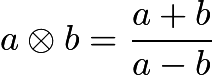$a\otimes b = \dfrac{a + b}{a - b}$, then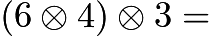$(6\otimes 4)\otimes 3 =$$\text{(A)}\ 4 \qquad \text{(B)}\ 13 \qquad \text{(C)}\ 15 \qquad \text{(D)}\ 30 \qquad \text{(E)}\ 72$

Source: AMC8 2001

Nov 16: In this addition problem, each letter stands for a different digit.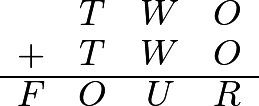$\setlength{\tabcolsep}{0.5mm}\begin{array}{cccc}&T & W & O\\ + &T & W & O\\ \hline F& O & U & R\end{array}$

If$T = 7$ and the letter$O$ represents an even number, what is the only possible value for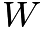$W$?$\textbf{(A)}\ 0 \qquad \textbf{(B)}\ 1 \qquad \textbf{(C)}\ 2\qquad \textbf{(D)}\ 3\qquad \textbf{(E)}\ 4$

Source: AMC8 2003

Nov 17: For any positive integer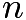$n$, define$\boxed{n}$ to be the sum of the positive factors of$n$. For example,$\boxed{6} = 1 + 2 + 3 + 6 = 12$. Find$\boxed{\boxed{11}}$ .$\mathrm{(A)}\ 13 \qquad \mathrm{(B)}\ 20 \qquad \mathrm{(C)}\ 24 \qquad \mathrm{(D)}\ 28 \qquad \mathrm{(E)}\ 30$

Source: AMC8 2007

Nov 18: In the product shown,$\text{B}$ is a digit. The value of$\text{B}$ is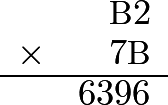$$\begin{array}{rr} &\text{B}2 \\ \times &7\text{B} \\ \hline &6396 \\ \end{array}$$$\text{(A)}\ 3 \qquad \text{(B)}\ 5 \qquad \text{(C)}\ 6 \qquad \text{(D)}\ 7 \qquad \text{(E)}\ 8$

Source: AMC8 1986

Nov 19:  If$\text{A}$ and$\text{B}$ are nonzero digits, then the number of digits (not necessarily different) in the sum of the three whole numbers is$$\begin{tabular}[t]{cccc} 9 & 8 & 7 & 6 \\ & A & 3 & 2 \\ & & B & 1 \\ \hline \end{tabular}$$$\text{(A)}\ 4 \qquad \text{(B)}\ 5 \qquad \text{(C)}\ 6 \qquad \text{(D)}\ 9 \qquad \text{(E)}\ \text{depends on the values of A and B}$

Source: AMC8 1987
Nov 20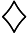$\diamondsuit$ and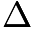$\Delta$ are whole numbers and$\diamondsuit \times \Delta =36$. The largest possible value of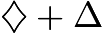$\diamondsuit + \Delta$ is$\text{(A)}\ 12 \qquad \text{(B)}\ 13 \qquad \text{(C)}\ 15 \qquad \text{(D)}\ 20\ \qquad \text{(E)}\ 37$

Source: AMC8 1988

Week of Nov 7 – Nov 13: This week’s problems are to do with distance and speed. Click GoogleForm to submit answers.

Answers: C, A, D, D, B, C, E

Nov 7: On a dark and stormy night Snoopy suddenly saw a flash of lightning. Ten seconds later he heard the sound of thunder. The speed of sound is 1088 feet per second and one mile is 5280 feet. Estimate, to the nearest half-mile, how far Snoopy was from the flash of lightning.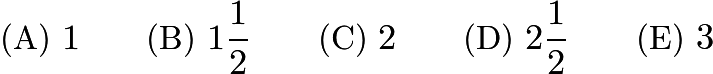$\text{(A)}\ 1 \qquad \text{(B)}\ 1\frac{1}{2} \qquad \text{(C)}\ 2 \qquad \text{(D)}\ 2\frac{1}{2} \qquad \text{(E)}\ 3$

Source: AMC8 2001

Nov 8: Homer began peeling a pile of 44 potatoes at the rate of 3 potatoes per minute. Four minutes later Christen joined him and peeled at the rate of 5 potatoes per minute. When they finished, how many potatoes had Christen peeled?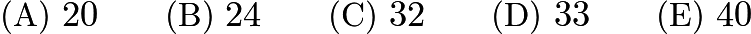$\text{(A)}\ 20 \qquad \text{(B)}\ 24 \qquad \text{(C)}\ 32 \qquad \text{(D)}\ 33 \qquad \text{(E)}\ 40$

Source: AMC8 2001

Nov 9: Joe had walked half way from home to school when he realized he was late. He ran the rest of the way to school. He ran 3 times as fast as he walked. Joe took 6 minutes to walk half way to school. How many minutes did it take Joe to get from home to school?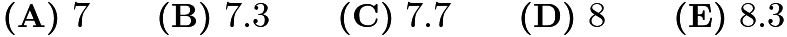$\textbf{(A)}\ 7\qquad\textbf{(B)}\ 7.3\qquad\textbf{(C)}\ 7.7\qquad\textbf{(D)}\ 8\qquad\textbf{(E)}\ 8.3$

Source: AMC8 2005

Nov 10: Cassie leaves Escanaba at 8:30 AM heading for Marquette on her bike. She bikes at a uniform rate of 12 miles per hour. Brian leaves Marquette at 9:00 AM heading for Escanaba on his bike. He bikes at a uniform rate of 16 miles per hour. They both bike on the same 62-mile route between Escanaba and Marquette. At what time in the morning do they meet?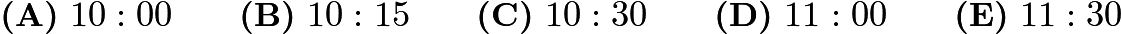$\textbf{(A)}\ 10: 00\qquad\textbf{(B)}\ 10: 15\qquad\textbf{(C)}\ 10: 30\qquad\textbf{(D)}\ 11: 00\qquad\textbf{(E)}\ 11: 30$

Source: AMC8 2006

Nov 11:  The students in Mrs. Reed’s English class are reading the same 760-page novel. Three friends, Alice, Bob and Chandra, are in the class. Alice reads a page in 20 seconds, Bob reads a page in 45 seconds and Chandra reads a page in 30 seconds.

If Bob and Chandra both read the whole book, Bob will spend how many more seconds reading than Chandra?$\textbf{(A)}\ 7,600\qquad\textbf{(B)}\ 11,400\qquad\textbf{(C)}\ 12,500\qquad\textbf{(D)}\ 15,200\qquad\textbf{(E)}\ 22,800$

Source: AMC8 2006

Nov 12: The students in Mrs. Reed’s English class are reading the same 760-page novel. Three friends, Alice, Bob and Chandra, are in the class. Alice reads a page in 20 seconds, Bob reads a page in 45 seconds and Chandra reads a page in 30 seconds.

Chandra and Bob, who each have a copy of the book, decide that they can save time by “team reading” the novel. In this scheme, Chandra will read from page 1 to a certain page and Bob will read from the next page through page 760, finishing the book. When they are through they will tell each other about the part they read. What is the last page that Chandra should read so that she and Bob spend the same amount of time reading the novel?$\textbf{(A)}\ 425\qquad\textbf{(B)}\ 444\qquad\textbf{(C)}\ 456\qquad\textbf{(D)}\ 484\qquad\textbf{(E)}\ 506$

Source: AMC8 2006
Nov 13: The students in Mrs. Reed’s English class are reading the same 760-page novel. Three friends, Alice, Bob and Chandra, are in the class. Alice reads a page in 20 seconds, Bob reads a page in 45 seconds and Chandra reads a page in 30 seconds.

Before Chandra and Bob start reading, Alice says she would like to team read with them. If they divide the book into three sections so that each reads for the same length of time, how many seconds will each have to read?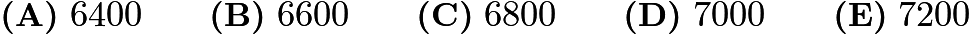$\textbf{(A)}\ 6400\qquad\textbf{(B)}\ 6600\qquad\textbf{(C)}\ 6800\qquad\textbf{(D)}\ 7000\qquad\textbf{(E)}\ 7200$

Source: AMC8 2006

Week of Oct 31 – Nov 6.  This week’s focus is on circles. Click GoogleForm to submit answers.

Answers: D, B, B, B, C, C, D

Oct 31: The area of the smallest square that will contain a circle of radius 4 is$\text{(A)}\ 8 \qquad \text{(B)}\ 16 \qquad \text{(C)}\ 32 \qquad \text{(D)}\ 64 \qquad \text{(E)}\ 128$

Source: AMC8 1998

Nov 1: Circle$X$ has a radius of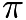$\pi$. Circle$Y$ has a circumference of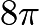$8 \pi$. Circle$Z$ has an area of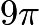$9 \pi$. List the circles in order from smallest to largest radius.$\textbf{(A)}\ X, Y, Z\qquad\textbf{(B)}\ Z, X, Y\qquad\textbf{(C)}\ Y, X, Z\qquad\textbf{(D)}\ Z, Y, X\qquad\textbf{(E)}\ X, Z, Y$

Source: AMC8 2006

Nov 2: Six pepperoni circles will exactly fit across the diameter of a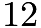$12$-inch pizza when placed. If a total of$24$ circles of pepperoni are placed on this pizza without overlap, what fraction of the pizza is covered by pepperoni?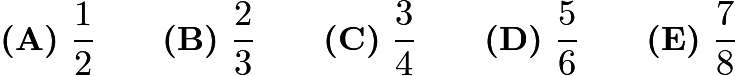$\textbf{(A)}\ \frac 12 \qquad\textbf{(B)}\ \frac 23 \qquad\textbf{(C)}\ \frac 34 \qquad\textbf{(D)}\ \frac 56 \qquad\textbf{(E)}\ \frac 78$

Source: AMC8 2010

Nov 3: A square and a circle have the same area. What is the ratio of the side length of the square to the radius of the circle?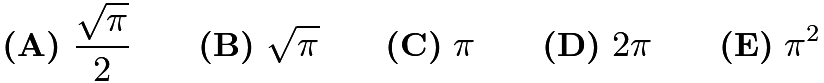$\textbf{(A)}\ \frac{\sqrt{\pi}}{2} \qquad\textbf{(B)}\ \sqrt{\pi} \qquad\textbf{(C)}\ \pi \qquad\textbf{(D)}\ 2\pi \qquad\textbf{(E)}\ \pi^{2}$

Source: AMC8 2010

Nov 4: Three circular arcs of radius 5 units bound the region shown. Arcs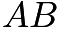$AB$ and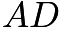$AD$are quarter-circles, and arc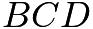$BCD$ is a semicircle. What is the area, in square units, of the region?$[asy] pair A,B,C,D; A = (0,0); B = (-5,5); C = (0,10); D = (5,5); draw(arc((-5,0),A,B,CCW)); draw(arc((0,5),B,D,CW)); draw(arc((5,0),D,A,CCW)); label($$\text{(A)}\ 25 \qquad \text{(B)}\ 10 + 5\pi \qquad \text{(C)}\ 50 \qquad \text{(D)}\ 50 + 5\pi \qquad \text{(E)}\ 25\pi$

Source: AMC8 2000

Nov 5: Diameter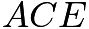$ACE$ is divided at$C$ in the ratio$2:3$. The two semicircles,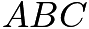$ABC$ and$CDE$, divide the circular region into an upper (shaded) region and a lower region. The ratio of the area of the upper region to that of the lower region is$[asy] pair A,B,C,D,EE; A = (0,0); B = (2,2); C = (4,0); D = (7,-3); EE = (10,0); fill(arc((2,0),A,C,CW)--arc((7,0),C,EE,CCW)--arc((5,0),EE,A,CCW)--cycle,gray); draw(arc((2,0),A,C,CW)--arc((7,0),C,EE,CCW)); draw(circle((5,0),5)); dot(A); dot(B); dot(C); dot(D); dot(EE); label($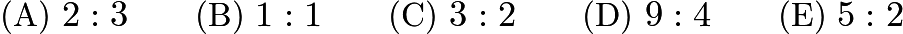$\text{(A)}\ 2:3 \qquad \text{(B)}\ 1:1 \qquad \text{(C)}\ 3:2 \qquad \text{(D)}\ 9:4 \qquad \text{(E)}\ 5:2$

Source: AMC8 1998

Nov 6: Two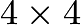$4 \times 4$ squares intersect at right angles, bisecting their intersecting sides, as shown. The circle’s diameter is the segment between the two points of intersection. What is the area of the shaded region created by removing the circle from the squares?$[asy] unitsize(6mm); draw(unitcircle); filldraw((0,1)--(1,2)--(3,0)--(1,-2)--(0,-1)--(-1,-2)--(-3,0)--(-1,2)--cycle,lightgray,black); filldraw(unitcircle,white,black); [/asy]$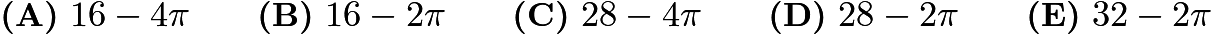$\textbf{(A)}\ 16-4\pi\qquad \textbf{(B)}\ 16-2\pi \qquad \textbf{(C)}\ 28-4\pi \qquad \textbf{(D)}\ 28-2\pi \qquad \textbf{(E)}\ 32-2\pi$

Source: AMC8 2004